# 5.2 Power functions and polynomial functions  (Page 8/19)

 Page 8 / 19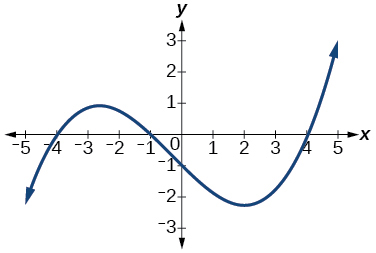3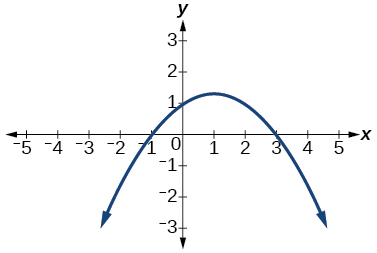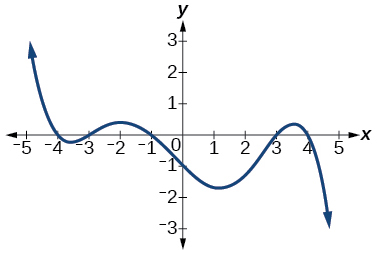5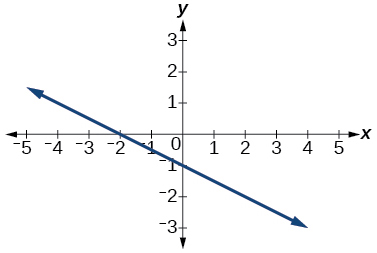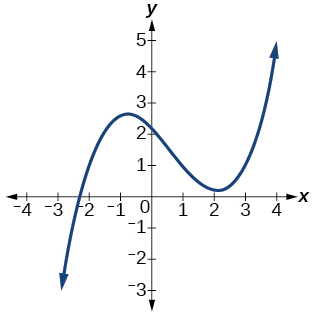3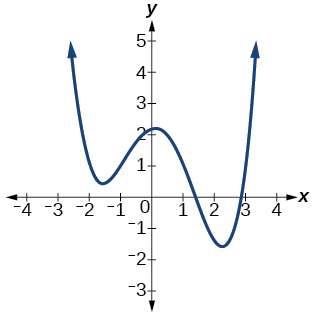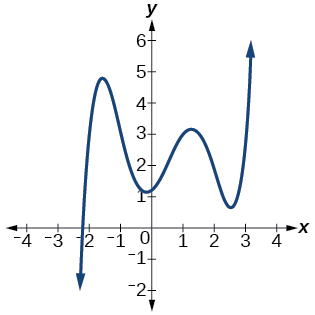5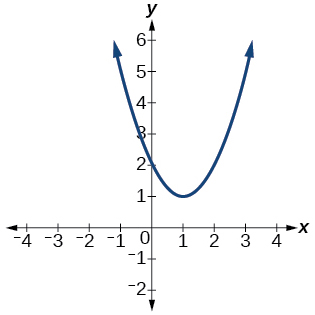For the following exercises, determine whether the graph of the function provided is a graph of a polynomial function. If so, determine the number of turning points and the least possible degree for the function.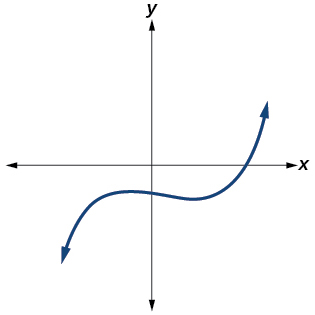Yes. Number of turning points is 2. Least possible degree is 3.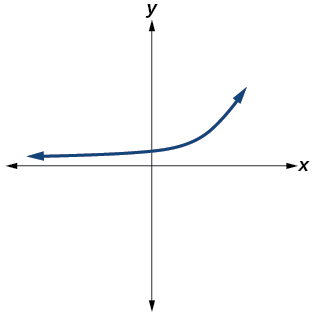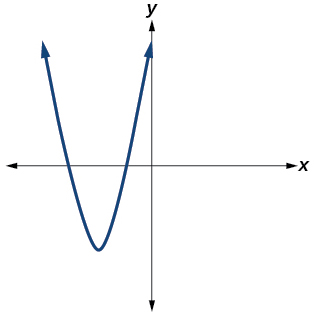Yes. Number of turning points is 1. Least possible degree is 2.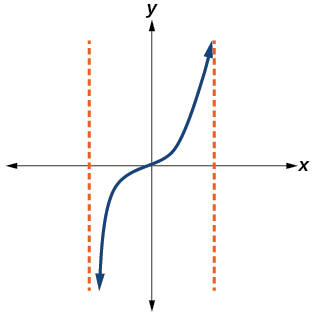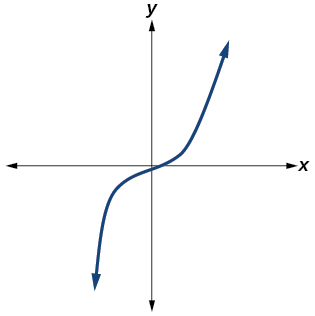Yes. Number of turning points is 0. Least possible degree is 1.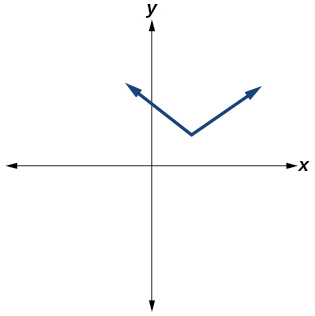No.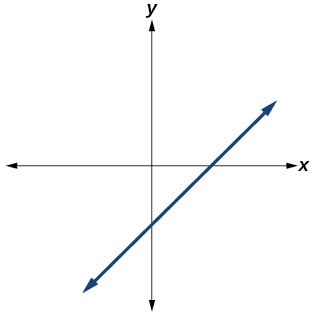Yes. Number of turning points is 0. Least possible degree is 1.

## Numeric

For the following exercises, make a table to confirm the end behavior of the function.

$f\left(x\right)=-{x}^{3}$

$f\left(x\right)={x}^{4}-5{x}^{2}$

$x$ $f\left(x\right)$
10 9,500
100 99,950,000
–10 9,500
–100 99,950,000

$\text{as}\text{\hspace{0.17em}}x\to -\infty ,\text{\hspace{0.17em}}\text{\hspace{0.17em}}f\left(x\right)\to \infty ,\text{\hspace{0.17em}}\text{as}\text{\hspace{0.17em}}x\to \infty ,\text{\hspace{0.17em}}f\left(x\right)\to \infty$

$f\left(x\right)={x}^{2}{\left(1-x\right)}^{2}$

$f\left(x\right)=\left(x-1\right)\left(x-2\right)\left(3-x\right)$

$x$ $f\left(x\right)$
10 –504
100 –941,094
–10 1,716
–100 1,061,106

$\text{as}\text{\hspace{0.17em}}x\to -\infty ,\text{\hspace{0.17em}}\text{\hspace{0.17em}}f\left(x\right)\to \infty ,\text{\hspace{0.17em}}\text{as}\text{\hspace{0.17em}}x\to \infty ,\text{\hspace{0.17em}}f\left(x\right)\to -\infty$

$f\left(x\right)=\frac{{x}^{5}}{10}-{x}^{4}$

## Technology

For the following exercises, graph the polynomial functions using a calculator. Based on the graph, determine the intercepts and the end behavior.

$f\left(x\right)={x}^{3}\left(x-2\right)$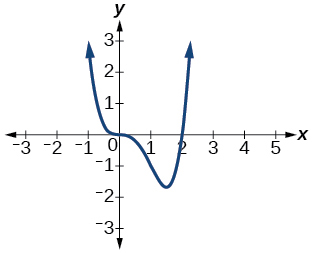The $\text{\hspace{0.17em}}y\text{-}$ intercept is The $\text{\hspace{0.17em}}x\text{-}$ intercepts are $\text{As}\text{\hspace{0.17em}}x\to -\infty ,\text{\hspace{0.17em}}\text{\hspace{0.17em}}f\left(x\right)\to \infty ,\text{\hspace{0.17em}}\text{as}\text{\hspace{0.17em}}x\to \infty ,\text{\hspace{0.17em}}f\left(x\right)\to \infty$

$f\left(x\right)=x\left(x-3\right)\left(x+3\right)$

$f\left(x\right)=x\left(14-2x\right)\left(10-2x\right)$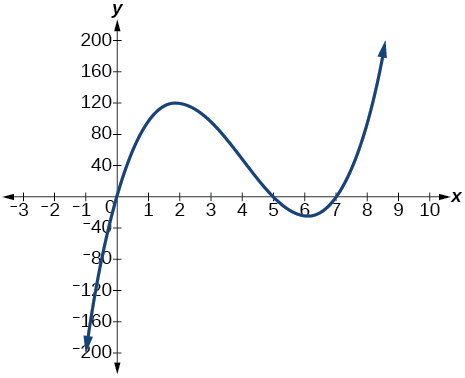The $\text{\hspace{0.17em}}y\text{-}$ intercept is $\text{\hspace{0.17em}}\left(0,0\right)$ . The $\text{\hspace{0.17em}}x\text{-}$ intercepts are $\text{As}\text{\hspace{0.17em}}x\to -\infty ,\text{\hspace{0.17em}}\text{\hspace{0.17em}}f\left(x\right)\to -\infty ,\text{\hspace{0.17em}}\text{as}\text{\hspace{0.17em}}x\to \infty ,\text{\hspace{0.17em}}f\left(x\right)\to \infty$

$f\left(x\right)=x\left(14-2x\right){\left(10-2x\right)}^{2}$

$f\left(x\right)={x}^{3}-16x$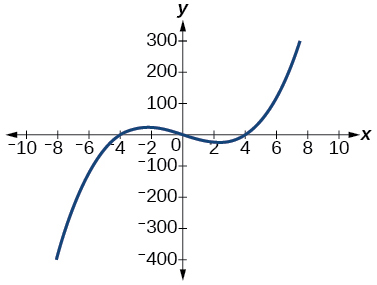The $\text{\hspace{0.17em}}y\text{-}$ intercept is The $\text{\hspace{0.17em}}x\text{-}$ intercept is $As\text{\hspace{0.17em}}x\to -\infty ,\text{\hspace{0.17em}}\text{\hspace{0.17em}}f\left(x\right)\to -\infty ,\text{\hspace{0.17em}}\text{as}\text{\hspace{0.17em}}x\to \infty ,\text{\hspace{0.17em}}f\left(x\right)\to \infty$

$f\left(x\right)={x}^{3}-27$

$f\left(x\right)={x}^{4}-81$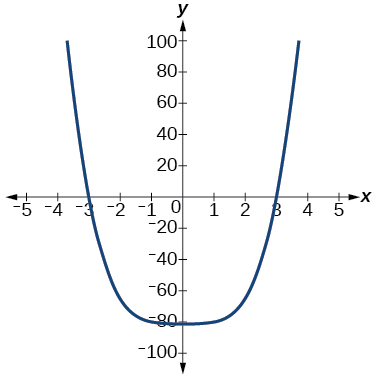The $\text{\hspace{0.17em}}y\text{-}$ intercept is The $\text{\hspace{0.17em}}x\text{-}$ intercept are $\text{As}\text{\hspace{0.17em}}x\to -\infty ,\text{\hspace{0.17em}}\text{\hspace{0.17em}}f\left(x\right)\to \infty ,\text{\hspace{0.17em}}\text{as}\text{\hspace{0.17em}}x\to \infty ,\text{\hspace{0.17em}}f\left(x\right)\to \infty$

$f\left(x\right)=-{x}^{3}+{x}^{2}+2x$

$f\left(x\right)={x}^{3}-2{x}^{2}-15x$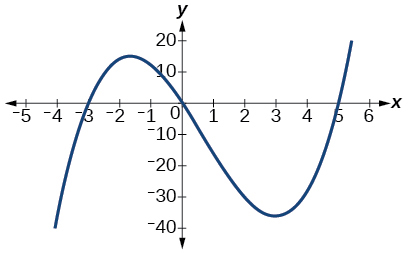The $\text{\hspace{0.17em}}y\text{-}$ intercept is The $\text{\hspace{0.17em}}x\text{-}$ intercepts are $\text{As}\text{\hspace{0.17em}}x\to -\infty ,\text{\hspace{0.17em}}\text{\hspace{0.17em}}f\left(x\right)\to -\infty ,\text{\hspace{0.17em}}\text{as}\text{\hspace{0.17em}}x\to \infty ,\text{\hspace{0.17em}}f\left(x\right)\to \infty$

$f\left(x\right)={x}^{3}-0.01x$

## Extensions

For the following exercises, use the information about the graph of a polynomial function to determine the function. Assume the leading coefficient is 1 or –1. There may be more than one correct answer.

The $\text{\hspace{0.17em}}y-$ intercept is $\text{\hspace{0.17em}}\left(0,-4\right).\text{\hspace{0.17em}}$ The $\text{\hspace{0.17em}}x-$ intercepts are $\text{\hspace{0.17em}}\left(-2,0\right),\text{\hspace{0.17em}}\left(2,0\right).\text{\hspace{0.17em}}$ Degree is 2.

End behavior: $\text{\hspace{0.17em}}\text{as}\text{\hspace{0.17em}}x\to -\infty ,\text{\hspace{0.17em}}\text{\hspace{0.17em}}f\left(x\right)\to \infty ,\text{\hspace{0.17em}}\text{as}\text{\hspace{0.17em}}x\to \infty ,\text{\hspace{0.17em}}f\left(x\right)\to \infty .$

$f\left(x\right)={x}^{2}-4$

The $\text{\hspace{0.17em}}y-$ intercept is $\text{\hspace{0.17em}}\left(0,9\right).\text{\hspace{0.17em}}$ The $\text{\hspace{0.17em}}x\text{-}$ intercepts are $\text{\hspace{0.17em}}\left(-3,0\right),\text{\hspace{0.17em}}\left(3,0\right).\text{\hspace{0.17em}}$ Degree is 2.

End behavior: $\text{\hspace{0.17em}}\text{as}\text{\hspace{0.17em}}x\to -\infty ,\text{\hspace{0.17em}}\text{\hspace{0.17em}}f\left(x\right)\to -\infty ,\text{\hspace{0.17em}}\text{as}\text{\hspace{0.17em}}x\to \infty ,\text{\hspace{0.17em}}f\left(x\right)\to -\infty .$

The $\text{\hspace{0.17em}}y-$ intercept is $\text{\hspace{0.17em}}\left(0,0\right).\text{\hspace{0.17em}}$ The $\text{\hspace{0.17em}}x-$ intercepts are $\text{\hspace{0.17em}}\left(0,0\right),\text{\hspace{0.17em}}\left(2,0\right).\text{\hspace{0.17em}}$ Degree is 3.

End behavior: $\text{\hspace{0.17em}}\text{as}\text{\hspace{0.17em}}x\to -\infty ,\text{\hspace{0.17em}}\text{\hspace{0.17em}}f\left(x\right)\to -\infty ,\text{\hspace{0.17em}}\text{as}\text{\hspace{0.17em}}x\to \infty ,\text{\hspace{0.17em}}f\left(x\right)\to \infty .$

$f\left(x\right)={x}^{3}-4{x}^{2}+4x$

The $\text{\hspace{0.17em}}y-$ intercept is $\text{\hspace{0.17em}}\left(0,1\right).\text{\hspace{0.17em}}$ The $\text{\hspace{0.17em}}x-$ intercept is $\text{\hspace{0.17em}}\left(1,0\right).\text{\hspace{0.17em}}$ Degree is 3.

End behavior: $\text{\hspace{0.17em}}\text{as}\text{\hspace{0.17em}}x\to -\infty ,\text{\hspace{0.17em}}\text{\hspace{0.17em}}f\left(x\right)\to \infty ,\text{\hspace{0.17em}}\text{as}\text{\hspace{0.17em}}x\to \infty ,\text{\hspace{0.17em}}f\left(x\right)\to -\infty .$

The $\text{\hspace{0.17em}}y-$ intercept is $\text{\hspace{0.17em}}\left(0,1\right).\text{\hspace{0.17em}}$ There is no $\text{\hspace{0.17em}}x-$ intercept. Degree is 4.

End behavior: $\text{\hspace{0.17em}}\text{as}\text{\hspace{0.17em}}x\to -\infty ,\text{\hspace{0.17em}}\text{\hspace{0.17em}}f\left(x\right)\to \infty ,\text{\hspace{0.17em}}\text{as}\text{\hspace{0.17em}}x\to \infty ,\text{\hspace{0.17em}}f\left(x\right)\to \infty .$

$f\left(x\right)={x}^{4}+1$

## Real-world applications

For the following exercises, use the written statements to construct a polynomial function that represents the required information.

An oil slick is expanding as a circle. The radius of the circle is increasing at the rate of 20 meters per day. Express the area of the circle as a function of $\text{\hspace{0.17em}}d,\text{\hspace{0.17em}}$ the number of days elapsed.

A cube has an edge of 3 feet. The edge is increasing at the rate of 2 feet per minute. Express the volume of the cube as a function of $\text{\hspace{0.17em}}m,\text{\hspace{0.17em}}$ the number of minutes elapsed.

$V\left(m\right)=8{m}^{3}+36{m}^{2}+54m+27$

A rectangle has a length of 10 inches and a width of 6 inches. If the length is increased by $\text{\hspace{0.17em}}x\text{\hspace{0.17em}}$ inches and the width increased by twice that amount, express the area of the rectangle as a function of $\text{\hspace{0.17em}}x.$

An open box is to be constructed by cutting out square corners of $\text{\hspace{0.17em}}x-$ inch sides from a piece of cardboard 8 inches by 8 inches and then folding up the sides. Express the volume of the box as a function of $\text{\hspace{0.17em}}x.$

$V\left(x\right)=4{x}^{3}-32{x}^{2}+64x$

A rectangle is twice as long as it is wide. Squares of side 2 feet are cut out from each corner. Then the sides are folded up to make an open box. Express the volume of the box as a function of the width ( $x$ ).

how do I set up the problem?
what is a solution set?
Harshika
find the subring of gaussian integers?
Rofiqul
hello, I am happy to help!
Abdullahi
hi mam
Mark
find the value of 2x=32
divide by 2 on each side of the equal sign to solve for x
corri
X=16
Michael
Want to review on complex number 1.What are complex number 2.How to solve complex number problems.
Beyan
yes i wantt to review
Mark
use the y -intercept and slope to sketch the graph of the equation y=6x
how do we prove the quadratic formular
Darius
hello, if you have a question about Algebra 2. I may be able to help. I am an Algebra 2 Teacher
thank you help me with how to prove the quadratic equation
Seidu
may God blessed u for that. Please I want u to help me in sets.
Opoku
what is math number
4
Trista
x-2y+3z=-3 2x-y+z=7 -x+3y-z=6
can you teacch how to solve that🙏
Mark
Solve for the first variable in one of the equations, then substitute the result into the other equation. Point For: (6111,4111,−411)(6111,4111,-411) Equation Form: x=6111,y=4111,z=−411x=6111,y=4111,z=-411
Brenna
(61/11,41/11,−4/11)
Brenna
x=61/11 y=41/11 z=−4/11 x=61/11 y=41/11 z=-4/11
Brenna
Need help solving this problem (2/7)^-2
x+2y-z=7
Sidiki
what is the coefficient of -4×
-1
Shedrak
the operation * is x * y =x + y/ 1+(x × y) show if the operation is commutative if x × y is not equal to -1
An investment account was opened with an initial deposit of \$9,600 and earns 7.4% interest, compounded continuously. How much will the account be worth after 15 years?
lim x to infinity e^1-e^-1/log(1+x)
given eccentricity and a point find the equiationByBy OpenStaxBy Jonathan LongBy OpenStaxBy OpenStaxBy Jonathan LongBy OpenStaxBy Mike WolfBy Sheila LopezBy Robert MurphyBy Rhodes